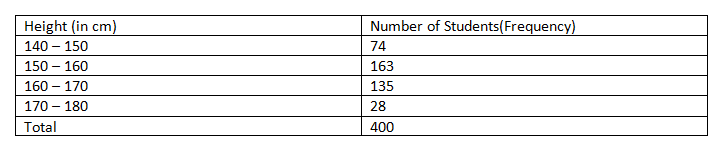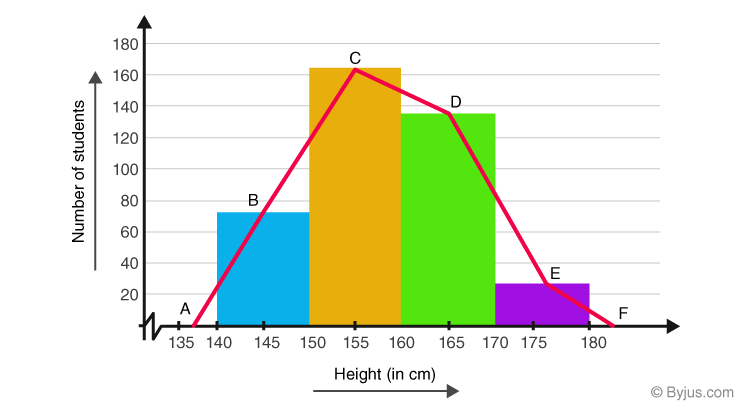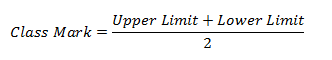# Frequency Polygons

A frequency polygon is almost identical to a histogram, which is used to compare sets of data or to display a cumulative frequency distribution. It uses a line graph to represent quantitative data.

Statistics deals with the collection of data and information for a particular purpose. The tabulation of each run for each ball in cricket gives the statistics of the game. Tables, graphs, pie-charts, bar graphs, histograms, polygons etc. are used to represent statistical data pictorially.

Frequency polygons are a visually substantial method of representing quantitative data and its frequencies. Let us discuss how to represent a frequency polygon.

## Steps to Draw Frequency Polygon

To draw frequency polygons, first we need to draw histogram and then follow the below steps:

• Step 1- Choose the class interval and mark the values on the horizontal axes
• Step 2- Mark the mid value of each interval on the horizontal axes.
• Step 3- Mark the frequency of the class on the vertical axes.
• Step 4- Corresponding to the frequency of each class interval, mark a point at the height in the middle of the class interval
• Step 5- Connect these points using the line segment.
• Step 6- The obtained representation is a frequency polygon.

Let us consider an example to understand this in a better way.

### Example

Example 1: In a batch of 400 students, the height of students is given in the following table. Represent it through a frequency polygon.Solution: Following steps are to be followed to construct a histogram from the given data:

• The heights are represented on the horizontal axes on a suitable scale as shown.
• The number of students is represented on the vertical axes on a suitable scale as shown.
• Now rectangular bars of widths equal to the class- size and the length of the bars corresponding to a frequency of the class interval is drawn.

ABCDEF represents the given data graphically in form of frequency polygon as:Frequency polygons can also be drawn independently without drawing histograms. For this, the midpoints of the class intervals known as class marks are used to plot the points.